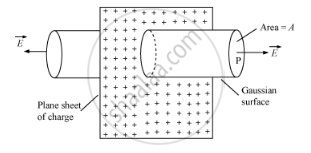# Use Gauss'S Law to Find the Electric Field Due to a Uniformly Charged Infinite Plane Sheet. What is the Direction of Field for Positive and Negative Charge Densities - Physics

Use Gauss's law to find the electric field due to a uniformly charged infinite plane sheet. What is the direction of field for positive and negative charge densities?

Use Gauss's theorem to find the electric field due to a uniformly charged infinitely large plane thin sheet with surface charge density σ.

#### Solution

Electric Field Due to an Infinite Plane Sheet of ChargeConsider an infinite thin plane sheet of positive charge with a uniform surface charge density σ on both sides of the sheet. Let P be the point at a distance a from the sheet at which the electric field is required. Draw a Gaussian cylinder of area of cross-section A through point P.

The electric flux crossing through the Gaussian surface,

Φ = E × Area of the circular caps of the cylinder

Since electric lines of force are parallel to the curved surface of the cylinder, the flux due to the electric field of the plane sheet of charge passes only through the two circular caps of the cylinder.

Φ = E × 2A … (i)

According to Gauss' Theorem,

phi=q/epsilon_0

Here, the charge enclosed by the Gaussian surface,

q = σA

:.phi=(sigmaA)/epsilon_0" ....(ii)"

From equations (i) and (ii), we get:

Exx2A=(sigmaA)/epsilon_0

 E=sigma/(2epsilon_0)

The direction of an electric field for positive charge density is in outward direction and perpendicular to the plane infinite sheet. And for the negative charge density the direction of the field is in inward direction and perpendicular to the sheet.

Concept: Uniformly Charged Infinite Plane Sheet and Uniformly Charged Thin Spherical Shell (Field Inside and Outside)
Is there an error in this question or solution?
2015-2016 (March) All India Set 2 C

Share